# Material Balance Equation in Reservoir Engineering

Material balance is a mathematic way to express mass conservation in a reservoir and a simple key principle is “what reservoir is produced must be replaced by other mass.

Volume Produced = Volume Replaced

Volume Produced comes from Gas Production, Oil Production, and Water Production.

Volume Replaced comes from volume expansion, water in flux and water/gas injection.

Figure 1 shows the relationship of the material balance.Figure 1 – Concept of Material Balance

Let’s take a look at each component of equation.

Volume Produced

Gas Production (rb) = Np (Rp – Rs) Bg

Oil Production (rb) = Np Bo

Water Production (rb) = Wp Bw

Total Production = Np [Bo + (Rp – Rs) Bg ] + Wp Bw

Volume Expansion

Oil Expansion

Oil expansion consists of two parts. The first one is only oil expansion and the second one is solution gas expansion that happens when reservoir pressure is below a bubble point.

Oil expansion (rb) = N (Bo – Boi)

Solution gas expansion (rb) = N (Rsi – Rs) Bg

Total oil expansion (rb) = N [ (Bo – Boi) + (Rsi – Rs) Bg ]

Rock and Connate Water Expansion

Rock expansion (rb) = Vp ×Cf ×ΔP

Connate water expansion (rb) = Vp ×Swc× Cw ×ΔP

Total rock and connate water expansion (rb) = Vp ×(Cf + Swc× Cw)× ΔP

Pore Volume (Vp) = [ N× (1 + m) ×Boi ]÷ (1 – Swc)Initial Gas Cap Expansion

Initial gas cap volume (rb) = m ×N ×Boi

Current gas cap volume (rb) = m ×N ×Boi ×(Bg ÷ Bgi)

Gas cap expansion (rb) = Current gas cap volume – Initial gas cap volume

Gas cap expansion (rb) = m ×N ×Boi ×[(Bg ÷ Bgi)-1]

Expansion Term

Oil expansion term (Eo)

Eo = (Bo – Boi) + (Rsi – Rs) ×Bg

Rock and connate water expansion term (Ef,w)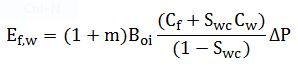Gas cap expansion term (Eg)

Eg = Boi ×[(Bg ÷ Bgi)-1]

With all expansion terms, total expansion can be mathematically expressed like this:

Total expansion (rb) = N (Eo + Efw + m Eg)

Aquifer Influx and Injection

Aquifer influx volume (rb) = We

Water injection volume (rb) = Wi×Bw

Gas injection volume (rb) = Gi×Bg

All relationships can be put in a material balance equation based on the following concept:The equation above is simplified by using an expansion term.The equation above can be rearranged like this: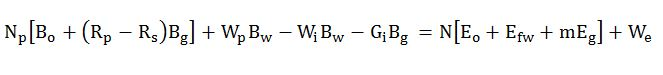Simplify a term like this:# F = N×Et + We

Where;

F = Net fluid production (Volume Produced – Volume Injection)

N = Oil in place

Et = Total expansion term (oil, water, gas cap and rock)

We = water influx

This simplified equation was presented by Havlena + Odeh (1963). With this equation form, graphical plots can be easily made and material balance evaluation is donemore easily and accurately.

# #1 form:The graphic can be drawn based on #1 form. The line should be a horizontal line and an intersection at y-axis is N (oil in place). Deviation from the horizontal line indicates adding or losing enery (Figure 2).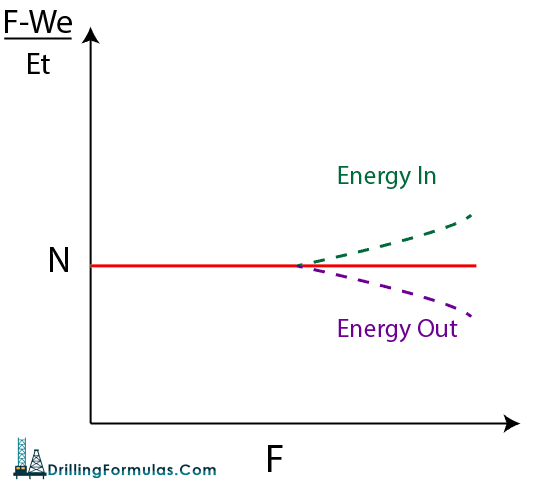Figure 2 – Graphic of Simplified Material Balance Form#1

# #2 form:

This is the form of a simplified material balance equation.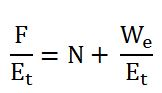A slope of the curve is 1 and the intersection on the y-axis is N. Deviation from the straight line indicates extra energy in or energy out (Figure 3).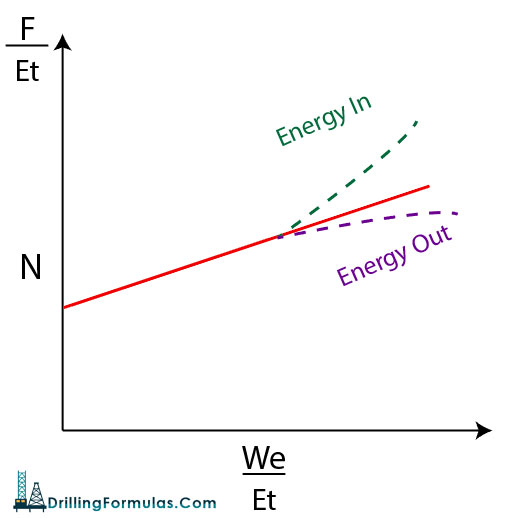Figure 3 – Graphic of Simplified Material Balance Form#2

Nomenclatures

N = oil initially in place (STOIIP) in reservoir (stb)

Np = cumulative oil production (stb)

Boi = oil volume factor at initial reservoir pressure (rb/stb)

Bo = oil volume factor at current reservoir pressure (rb/stb)

Rsi = solution GOR at initial reservoir pressure (scf/stb)

Rs = solution GOR at current reservoir pressure (scf/stb)

Rp = cumulative produced gas oil ratio (scf/stb)

G = gas volume initially in place (GIIP) in reservoir (scf)

m = ratio of initial gas cap volume to initial oil volume (rb/rb)

Bgi = gas volume factor at initial reservoir pressure (rb/scf)

Bg = gas volume factor at current reservoir pressure (rb/scf)

Swc = connate water saturation (fraction or %)

Cw = water compressibility (1/psi)

Cf = formation (rock) compressibility (1/psi)

Wp = cumulative water production (stb)

We= cumulative water influx from aquifer (rb)

Bw = water volume factor at initial reservoir pressure (rb/stb)

Wi = cumulative water injection (stb)

Gi = cumulative gas injection (scf)

Gp = cumulative gas production (scf)

Eg = gas expansion term (rb/stb)

Eo = oil expansion term (rb/stb)

Efw = formation and connate water expansion term (rb/stb)

References

Abhijit Y. Dandekar, 2013. Petroleum Reservoir Rock and Fluid Properties, Second Edition. 2 Edition. CRC Press.

L.P. Dake, 1983. Fundamentals of Reservoir Engineering, Volume 8 (Developments in Petroleum Science). New impression Edition. Elsevier Science.

Tarek Ahmed PhD PE, 2011. Advanced Reservoir Management and Engineering, Second Edition. 2 Edition. Gulf Professional Publishing.

Share the joy
Tagged , , , , . Bookmark the permalink.